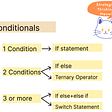# Balancing Braces

“Write a function isBalanced that returns true if a set of brackets is balanced.”

## Test cases

`isBalanced('()'); // trueisBalanced('()[]{}'); // trueisBalanced('([()]{})'); //trueisBalanced('('); // falseisBalanced(')'); // falseisBalanced('(]'); // falseisBalanced('([)]'); // false`

## Analysis

This is a parsing problem. It can be broken down into four outcomes:

1. Opener without closer e.g. ‘(’, or ‘()[’
2. Closer without opener e.g. ‘)’, or ‘()]’
3. Mismatched closer (aka mismatched opener) e.g. ‘(]’, or ‘([)]’
4. Balanced

The key point here is that this problem is harder than simply counting openers and closers because openers need to be matched with closers.

So to solve this problem we need to have a function that will return the opener corresponding to a closer.

The nature of the input data is that it consists of zero or more nests of brackets. Each nest is delineated by a closing bracket and is symmetrical first-opener to last-closer.

`() // The simplest nest.({}) // The second simplest nest.({}[]) // A nest containing two sub-nests.({}{[]}) // Another nest containing two sub-nests.({})() // Two nests.`

These nests can be of arbitrary complexity.

Importantly, a valid nest is closed in a carefully ordered fashion. No outer pair is closed until the inner pair is closed. This follows the principle of last-opened, first-closed, or last-in, first-out (LIFO). If we can find a data-structure that matches this behavior then we will avoid having to encode behavior in logic and instead leverage the innate behavior of the data structure.

The simplest data structure that exhibits this type of behavior is a stack. LIFO ordering is the raison d’être of that data structure.

Let’s work this through:

`Parsing Location            Stack        ({[]})                  <empty>    ^    ({[]})                    (      ^    ({[]})                    { TOP      ^                       ( BOTTOM    ({[]})                    [ TOP       ^                      {                              ( BOTTOM`

So we can see that the stack is constructing the expected closing tag order (but populated with the opening tags). This works because the opening and closing tags are mirror images of one another.

So the stack gives us a schema for what the closing tags should look like.

So when we find a closing bracket, we can look on the top of the stack to see if it is correct. We no longer need the top of the stack after checking it, so we discard it with the pop operation (instead of using peek).

So we have solved the “mismatched brackets” part of the problem. In other words we can detect when the nesting is invalid. This is the hardest part of the problem.

What remains is to count mismatches. This can be performed by straightforward counting. Seeing as we are already using a stack, we can leverage it to tell us this information: if anything is left on the stack at the end of parsing, then we have too many opening brackets.

For “too many closing brackets” then we need to return as soon as it is detected to avoid compensatory errors correcting the issue later in the parsing. Too many closing brackets can be detected when an attempt is made to pop the stack when it is already empty.

We are now ready to write our solution.

## Solution

`const OPEN = /^[\{\[\(]/;const CLOSE = /^[\}\]\)]/;const INVERT = {  '}': '{',   ']': '[',   ')': '(',};function isBalanced(str) {  var arr, curr, stack, opener;  arr = str.split('');  stack = []; // LIFO!  while (arr.length) {    curr = arr.shift();     if (OPEN.test(curr)) {      stack.push(curr); // Assume openers OK (until end of `arr`).     } else if (CLOSE.test(curr)) {      if (!(stack.length)) {        return false; // Closer without opener in current nest.      }      // `opener` opened the current pair.       // `pop` reveals the previous opener!      opener = stack.pop(curr);      if (opener !== INVERT[curr]) {        return false; // “Mismatched closer”.      }    }  }  // “Missing closer” if anything  // left on the stack, otherwise,   // balanced.  return !(stack.length); }`

My name is Ben Aston and thank you for reading my post today. I am a London-based JavaScript consultant, available for short-term consultancy globally. I can be contacted at ben@bj.ma.

If you would like to further develop your knowledge of JavaScript you might be interested in my hands-on training course. Full details can be found online at www.learnjavascript.london.

If you enjoyed this piece, please let me know by clicking the “recommend” button below.

You may also be interested in my video entitled An Introduction to Modern JavaScript.

--

--

--

## More from Ben Aston

London, England

Love podcasts or audiobooks? Learn on the go with our new app.London, England

## Conditionals in JavaScript, Meow!## Axios Throttle## Special Concepts about JavaScript & MongoDB